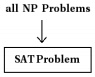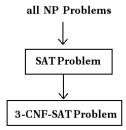SAT (Boolean Formula Satisfiability Problem)

# SAT (Boolean Formula Satisfiability Problem) - Theory of Computation - Computer Science Engineering (CSE)

Part IV. SAT (Boolean Formula Satisfiability Problem)

7 SAT

Satisfiability
An example for a boolean formula is
(¬x1 ∪ x2) ∩ (x1 ∪ ¬x2) ∩ (¬x2 ∪ x3) ∩ (¬x∪ ¬x2 ∪ x3).
This formula is in conjunctive normal form (CNF) (intersection of unions).
This boolean formula is satisfiable if there is some assignment of the values 0 and 1 to its variables that causes it to
evaluate to 1 (true).
If we put, x= 0, x2 = 0, x3 = 1, we can see that the above formula evaluates to 1 (true).
This means above formula is satisfiable.

Consider the boolean formula,
(x1 ∪ x2) ∩ (¬x1 ∪ ¬x2)
If we put x1 = 0, x2 = 0, this formula evaluates to 0. If we put x1 = 0, x2 = 1, this formula evaluates to 0. If we put
x1 = 1, x= 0, this formula evaluates to 0. If we put x1 = 1, x2 = 1, this formula evaluates to 0. This means for any values assigned to the variables the above boolean formula always evaluate to 0 (false).
This means the formula is not satisfiable.

SAT Problem
SAT (Boolean Formula Satisfiability) problem is defined as:
Is there a set of values for the boolean variables that assigns the value 1 (true) to the boolean expression given in CNF?

8 Cook’s Theorem
Cook’s theorem states that
satisfiability of boolean formulas (SAT problem) is NP-Complete.

SAT Problem is NP - Complete
We know that a problem is in class NP-Complete if: it is in NP and it is NP Hard.

Theorem:
Satisfiability of boolean formulas is NP-Complete.

Proof:
Step 1: Prove that SAT problem is in class NP.
A boolean variable can have two possible values. Hence, for an n variable boolean expression in CNF, there are 2n possible combinations of values of these variables.
If we want to check whether a boolean formula is satisfiable, we need to apply each combination to the formula until we get a 1 as the result of evaluation. In the worst case, we need to apply all the 2possible combinations.
Consider the formula, (¬x1 ∪ x2) ∩ (x1 ∪ ¬x2) ∩ (¬x2 ∪ x3) ∩ (¬x1 ∪ ¬x2 ∪ x3). There are 3 variables. Each variable can have two possible values, 0 or 1. There are 23 combinations. So the time complexity of this problem is Θ(2n). (not polynomial time) If we are given a certificate containing a satisfiable assignment for a formula, then we can easily verify it in polynomial time.
For example, for the above formula, if we are given a certificate saying that if x1 = 0, x2 = 0, x= 1, then just applying these values to the formula, we get,
(¬x1 ∪ x2) ∩ (x1 ∪ ¬x2) ∩ (¬x2 ∪ x3) ∩ (¬x1 ∪ ¬x2 ∪ x3)
=(¬0 ∪ 0) ∩ (0 ∪ ¬0) ∩ (¬0 ∪ 1) ∩ (¬0 ∪ ¬0 ∪ 1)
=(1 ∪ 0) ∩ (0 ∪ 1) ∩ (1 ∪ 1) ∩ (1 ∪ 1 ∪ 1)
=1 ∩ 1 ∩ 1 ∩ 1=1
This can be done in linear time.
So the SAT problem is in class NP.

Step 2: Prove that SAT problem is NP-hard.
We know that a problem is NP-Hard, if every problem in NP can be reduced to this problem in polynomial time.A lot of details are involved in this proof. [This proof is beyond the scope of this class].
[Refer the text book ’Introduction to the Theory of computation’ by Michael Sipser - Chapter 7- Theorem 7.37]

3-CNF Satisfiability Problem (3-CNF-SAT)

3-CNF
We know that a boolean formula is in conjunctive normal form(CNF) if it is expressed as an AND of clauses, each clause is the OR of one or more variables.
Then, a formula is in 3-conjunctive normal form (3-CNF) is each clause has exactly three distinct literals.
For example, the following boolean formula is in 3-CNF.
(x1 ∪ ¬x1 ∪ ¬x2) ∩ (x3 ∪ x2 ∪ x4) ∩ (¬x1 ∪ ¬x3 ∪ ¬x4) ∩ (x2 ∪ x1 ∪ ¬x3)
The clauses in the above formula contain exactly 3 literals. The formula is in 3-CNF.

3-CNF Satisfiability Problem
3-CNF-SAT problem is defined as:
Is there a set of values for the boolean variables that assigns the value 1 (true) to the boolean expression given in 3-CNF?
3-CNF Satisfiability Problem is NP-complete
We know that a problem is in class NP-Complete if: it is in NP and it is NP Hard.

Theorem:
Satisfiability of boolean formulas in 3-CNF is NP-complete.

Proof:
Step 1: Prove that 3-CNF-SAT is in class NP.
A boolean variable can have two possible values. Hence, for an n variable boolean expression in CNF, there are 2n possible combinations of values of these variables.
If we want to check whether a boolean formula is satisfiable, we need to apply each combination to the formula until we
get a 1 as the result of evaluation. In the worst case, we need to apply all the 2n possible combinations.
Consider the 3-CNF formula, (x1 ∪ ¬x1 ∪ ¬x2) ∩ (x3 ∪ x2 ∪ x4) ∩ (¬x1 ∪ ¬x3 ∪ ¬x4) ∩ (x2 ∪ x1 ∪ ¬x3). There are 4 variables. Each variable can have two possible values, 0 or 1. There are 24 combinations.So the time complexity of this problem is Θ(2n). (not polynomial time) If we are given a certificate containing a satisfiable assignment for a formula, then we can easily verify it in polynomial time.
For example, for the above formula, if we are given a certificate saying that if x1 = 0, x2 = 1, x3 = 0, x4 = 1, then
just applying these values to the formula, we get,

(x1 ∪ ¬x1 ∪ ¬x2) ∩ (x3 ∪ x2 ∪ x4) ∩ (¬x1 ∪ ¬x3 ∪ ¬x4) ∩ (x2 ∪ x1 ∪ ¬x3)
=(0 ∪ ¬0 ∪ ¬1) ∩ (0 ∪ 1 ∪ 1) ∩ (¬0 ∪ ¬0 ∪ ¬1) ∩ (1 ∪ 0 ∪ ¬0)
=(0 ∪ 1 ∪ 0) ∩ (0 ∪ 1 ∪ 1) ∩ (1 ∪ 1 ∪ 0) ∩ (1 ∪ 0 ∪ 1)
=1 ∩ 1 ∩ 1 ∩ 1 ∩ 1=1
This can be done in linear time.
So the 3-CNF-SAT problem is in class NP.

Step 2: Prove that 3-CNF-SAT problem is NP-hard.
We know that a problem is NP-Hard, if every problem in NP can be reduced to this problem in polynomial time.
The approach we used is as follows:
In the previous section, we already proved that all NP problems can be reduced to SAT. Then, if we can reduce SAT to 3-CNF-SAT in polynomial time, we can say that 3-CNF-SAT is NP-hard.We learned in the subject, ’logic design’ that any boolean formula can be expressed in CNF. Then, a boolean formula
in CNF can be rewritten in 3-CNF.
Consider a boolean formula as follows:
a ∪ b ∪ c ∪ d
This can be converted to 3-CNF as,
(a ∪ b ∪ x) ∩ (c ∪ d ∪ x)
Thus any boolean formula can be transformed to a 3-CNF boolean formula. This can be done using De Morgan’s laws.
That means SAT problem can be reduced to 3-CNF-SAT problem. That is, 3-CNF-SAT is NP-hard.

In step 1, we proved that 3-CNF-SAT is in NP.
In step 2, we proved that 3-CNF-SAT is NP-hard.
So, 3-CNF-SAT is an NP-complete problem.

The document SAT (Boolean Formula Satisfiability Problem) | Theory of Computation - Computer Science Engineering (CSE) is a part of the Computer Science Engineering (CSE) Course Theory of Computation.
All you need of Computer Science Engineering (CSE) at this link: Computer Science Engineering (CSE)

## Theory of Computation

18 videos|56 docs|44 tests

## FAQs on SAT (Boolean Formula Satisfiability Problem) - Theory of Computation - Computer Science Engineering (CSE)

 1. What is the SAT problem in computer science engineering?Ans. The SAT problem, also known as the Boolean Formula Satisfiability Problem, is a fundamental problem in computer science engineering. It involves determining whether there exists an assignment of truth values to the variables in a given Boolean formula that satisfies the formula as a whole.
 2. How is the SAT problem relevant to computer science engineering?Ans. The SAT problem is highly relevant to computer science engineering as it has numerous applications in different areas of the field. It is used in designing and verifying hardware circuits, optimizing software, solving constraint satisfaction problems, and even in artificial intelligence and automated reasoning.
 3. What is the complexity of solving the SAT problem in computer science engineering?Ans. The SAT problem is classified as an NP-complete problem, which means that it is one of the most challenging problems to solve efficiently. It is believed that there is no known algorithm that can solve SAT in polynomial time for all possible inputs. However, various algorithms and heuristics have been developed to tackle SAT instances effectively.
 4. What are some common techniques used to solve the SAT problem?Ans. There are several techniques commonly used to solve the SAT problem in computer science engineering. These include backtracking algorithms, such as the Davis-Putnam-Logemann-Loveland (DPLL) algorithm, which systematically explores the search space of possible truth assignments. Other techniques include conflict-driven clause learning, unit propagation, and variable and value ordering heuristics.
 5. What are the practical implications of solving the SAT problem in computer science engineering?Ans. Solving the SAT problem has significant practical implications in computer science engineering. It enables the verification of hardware and software designs, aids in solving complex optimization problems, and plays a crucial role in automated reasoning and formal verification. Additionally, SAT solvers are used in various industries, including electronic design automation, software engineering, and operations research, to optimize and improve system performance.

## Theory of Computation

18 videos|56 docs|44 testsExplore Courses for Computer Science Engineering (CSE) examSignup to see your scores go up within 7 days! Learn & Practice with 1000+ FREE Notes, Videos & Tests.
10M+ students study on EduRev
Track your progress, build streaks, highlight & save important lessons and more!
Related Searches

,

,

,

,

,

,

,

,

,

,

,

,

,

,

,

,

,

,

,

,

,

;